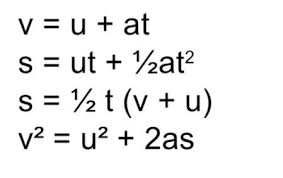# Physics formula for semester 1

Physics formula :-What is instantaneous velocity?
Instantaneous velocity is the velocity of an object in motion at a specific point in time. This is determined similarly to average velocity, but we narrow the period of time so that it approaches zero. If an object has a standard velocity over a period of time, its average and instantaneous velocities may be the same.
A goods elevator moves with 5 m/s upwards. Suddenly, at a height of 30 m, the rope that holds the elevator snaps and the elevator is falling down. a) How much time does the elevator need to crash into the ground? b) What is its velocity then? c) What is the total falling height? (Hints: use the complete formula for the free fall, i.e. with starting velocity and starting position.